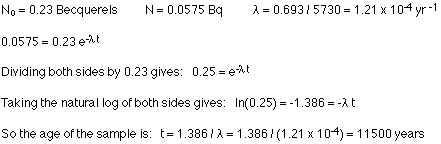8-11-99

### The nucleus

When we looked at the atom from the point of view of quantum mechanics, we treated the nucleus as a positive point charge and focused on what the electrons were doing. In many cases, such as in chemical reactions, that's all that matters; in other cases, such as radioactivity, or for nuclear reactions, what happens in the nucleus is critical, and the electrons can be ignored.

A nucleus consists of a bunch of protons and neutrons; these are known as nucleons. Each nucleus can be characterized by two numbers: A, the atomic mass number, which is the total number of nucleons; and Z, the atomic number, representing the number of protons. Any nucleus can be written in a form like this: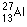where Al is the element (aluminum in this case), the 27 is the atomic mass number (the number of neutrons plus the number of protons), and the 13 is Z, the atomic number, the number of protons.

How big is a nucleus? We know that atoms are a few angstroms, but most of the atom is empty space. The nucleus is much smaller than the atom, and is typically a few femtometers. The nucleus can be thought of as a bunch of balls (the protons and neutrons) packed into a sphere, with the radius of the sphere being approximately: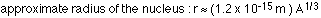### The strong nuclear force

What holds the nucleus together? The nucleus is tiny, so the protons are all very close together. The gravitational force attracting them to each other is much smaller than the electric force repelling them, so there must be another force keeping them together. This other force is known as the strong nuclear force; it works only at small distances. The strong nuclear force is a very strong attractive force for protons and neutrons separated by a few femtometers, but is basically negligible for larger distances.

The tug-of-war between the attractive force of the strong nuclear force and the repulsive electrostatic force between protons has interesting implications for the stability of a nucleus. Atoms with very low atomic numbers have about the same number of neutrons and protons; as Z gets larger, however, stable nuclei will have more neutrons than protons. Eventually, a point is reached beyond which there are no stable nuclei: the bismuth nucleus with 83 protons and 126 neutrons is the largest stable nucleus. Nuclei with more than 83 protons are all unstable, and will eventually break up into smaller pieces; this is known as radioactivity.

### Nuclear binding energy and the mass defect

A neutron has a slightly larger mass than the proton. These are often given in terms of an atomic mass unit, where one atomic mass unit (u) is defined as 1/12th of the mass of a carbon-12 atom.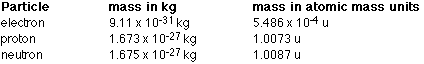Something should probably strike you as being a bit odd here. The carbon-12 atom has a mass of 12.000 u, and yet it contains 12 objects (6 protons and 6 neutrons) that each have a mass greater than 1.000 u. The fact is that these six protons and six neutrons have a larger mass when they're separated than when they're bound together into a carbon-12 nucleus.

This is true for all nuclei, that the mass of the nucleus is a little less than the mass of the individual neutrons and protons. This missing mass is known as the mass defect, and is essentially the equivalent mass of the binding energy.

Einstein's famous equation relates energy and mass:If you convert some mass to energy, Einstein's equation tells you how much energy you get. In any nucleus there is some binding energy, the energy you would need to put in to split the nucleus into individual protons and neutrons. To find the binding energy, then, all you need to do is to add up the mass of the individual protons and neutrons and subtract the mass of the nucleus:The binding energy is then: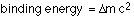In a typical nucleus the binding energy is measured in MeV, considerably larger than the few eV associated with the binding energy of electrons in the atom. Nuclear reactions involve changes in the nuclear binding energy, which is why nuclear reactions give you much more energy than chemical reactions; those involve changes in electron binding energies.

Many nuclei are radioactive. This means they are unstable, and will eventually decay by emitting a particle, transforming the nucleus into another nucleus, or into a lower energy state. A chain of decays takes place until a stable nucleus is reached.

During radioactive decay, principles of conservation apply. Some of these we've looked at already, but the last is a new one:

• conservation of energy
• conservation of momentum (linear and angular)
• conservation of charge
• conservation of nucleon number

Conservation of nucleon number means that the total number of nucleons (neutrons + protons) must be the same before and after a decay.

There are three common types of radioactive decay, alpha, beta, and gamma. The difference between them is the particle emitted by the nucleus during the decay process.

### Alpha decay

In alpha decay, the nucleus emits an alpha particle; an alpha particle is essentially a helium nucleus, so it's a group of two protons and two neutrons. A helium nucleus is very stable.

An example of an alpha decay involves uranium-238: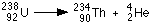The process of transforming one element to another is known as transmutation.

Alpha particles do not travel far in air before being absorbed; this makes them very safe for use in smoke detectors, a common household item.

### Beta decay

A beta particle is often an electron, but can also be a positron, a positively-charged particle that is the anti-matter equivalent of the electron. If an electron is involved, the number of neutrons in the nucleus decreases by one and the number of protons increases by one. An example of such a process is: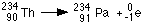In terms of safety, beta particles are much more penetrating than alpha particles, but much less than gamma particles.

### Gamma decay

The third class of radioactive decay is gamma decay, in which the nucleus changes from a higher-level energy state to a lower level. Similar to the energy levels for electrons in the atom, the nucleus has energy levels. The concepts of shells, and more stable nuclei having filled shells, apply to the nucleus as well.

When an electron changes levels, the energy involved is usually a few eV, so a visible or ultraviolet photon is emitted. In the nucleus, energy differences between levels are much larger, typically a few hundred keV, so the photon emitted is a gamma ray.

Gamma rays are very penetrating; they can be most efficiently absorbed by a relatively thick layer of high-density material such as lead.

A list of known nuclei and their properties can be found in the on-line chart of the nuclides .

Making a precise prediction of when an individual nucleus will decay is not possible; however, radioactive decay is governed by statistics, so it is very easy to predict the decay pattern of a large number of radioactive nuclei. The rate at which nuclei decay is proportional to N, the number of nuclei there are: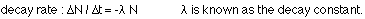Whenever the rate at which something occurs is proportional to the number of objects, the number of objects will follow an exponential decay. In other words, the equation telling you how many objects there are at a particular time looks like this:The decay constant is closely related to the half-life, which is the time it takes for half of the material to decay. Using the radioactive decay equation, it's easy to show that the half-life and the decay constant are related by: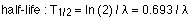The activity of a sample of radioactive material (i.e., a bunch of unstable nuclei) is measured in disintegrations per second, the SI unit for this being the becquerel.

### Understanding half-life using M&M's

Please note that M&M's are perfectly safe, and are not radioactive. M&M's can be used as a model of a sample of radioactive nuclei, however, because when they lie on a flat surface they can be in one of just two states - they can lie with the M up or with the M down. Let one of those states (M down, say) represent decayed nuclei.

With a package of M&M's, you can model a sample of decaying nuclei like this:

• step 1 - count the number of M&M's you have.
• step 2 - throw them onto a flat surface, and count the number of M&M's with M up. Remove all the M down M&M's from the sample.
• step 3 - repeat step 2 until you have no M&M's left.

Every time you throw the M&M's, you've gone through one more half-life. Here's the data from one trial: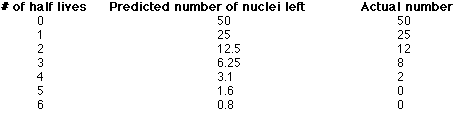The above is just a single trial; you should try it yourself to see what you get. This trial shows something interesting, however. When you have a large number of particles, they follow the predicted behavior very closely. When you only have a small number, the inherent randomness of the decay process is a little more obvious.